## Search form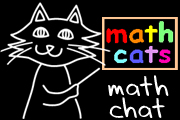# Picturing Mental Math

## By Wendy Petti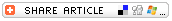Help students become more mathematically literate by giving them the tools for quick mental math.

When we use math in the "real world," we often need to come up with a quick number mentally in a few moments: How many more minutes until the end of class? How much will three tickets cost? What's my score now? We can help students become more mathematically literate by giving them tools and strategies for quick mental math.

The way we calculate mentally is not the way we calculate with paper and pencil. Students often are resistant to that notion; they try to use an invisible paper and a finger in the air or an imaginary pencil when "forced" to do mental math. It's easy to understand why they do that; it's what theyre accustomed to. It's not so easy to lead them into a new way of calculating, in which we take away not only their paper and pencil, but also their paper-and-pencil methods.

We can help students develop a mental math tool bag by modeling the tools concretely. Think of this transitional stage as mental math with training wheels. Now, let's take a look at some tools for picturing math linearly.

## NUMBER LINE

To help students develop a "mental number line," or a linear mental picture when adding or subtracting numbers, it can be effective to post a large number line (from 0 -100) at the front of the classroom and to use it regularly. Highlight all the multiples of 10; they serve as "anchors." Point to the number line with a yardstick to model how to jump along a number line to add or subtract two-digit numbers or skip-count by a given number.

When students are learning to skip-count as a strategy for learning multiplication tables, it helps to show them how they can "bridge" a ten: break a number into two chunks to jump to the nearest 10 and then jump beyond. For instance, skip-counting by 7s might look like this when students are starting out: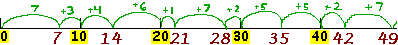The yardstick follows this pattern of jumps, touching on each anchor, as well as on each multiple of 7. At first, a teacher or student can "narrate" the jumps: "Jump 7; jump 3 and then 4 to 14; jump 6 and then 1 to 21; jump 7 to 28; jump 2 and then 5 to 35." After a few repetitions of narrated jumps, the yardstick jumping with bridging can be done silently as the class recites the 7s aloud. Eventually, the jumping can be done without bridging:The same approach can be applied to skip-counting by higher numbers, bridging tens as needed.

It can be helpful for each student to have a personal number line as well. You can use meter sticks with the tens highlighted for that purpose. However, we want to guide students away from counting by ones, as many students do in the lower grades. It helps to model jumping in chunks, as students use a finger to jump along a personal number line. Another strategy is to lay Cuisenaire rods along the meter stick.Â

## PARTIALLY NUMBERED LINE

Do we really need all the numbers labeled when using the number line as a tool, or do they get in the way? After considerable number line activity with the tens highlighted as anchors, switch to number lines with only the tens marked:With sufficient preparatory number line work, students should find it comfortable to skip-count, add, and subtract on a partially numbered line, using a finger or pencil to jump by tens and bridge the anchoring tens as needed. For example, to add 38 + 35, the student could start at 38 (by placing a pencil or finger to the left of 40), jump by 10 three times: 48, 58, 68 (placing the pencil to the left of 50, 60, and 70); jump 2 to reach 70; and then jump 3 more (ending about 1/3 of the distance between 70 and 80). The sparsely-numbered line makes it easier to think in terms of chunks.

## EMPTY NUMBER LINE

An "empty number line" is, just as the name suggests, a line without numbers. Students can add and subtract multi-digit numbers using an empty number line to record their steps, drawing jumps and writing a few numbers as needed.With the "jump strategy" used on empty number lines, students treat one number as a whole number and break the other number into manageable chunks. In order to use an empty number line or mental number line effectively, students need to be able to count by 10s, on and off the decade, and to jump across -- or bridge -- tens. Let's look at how those two skills are applied in the samples. In the addition example, the student begins with 56 near the left end of the line, jumps two tens, and then breaks the 7 into 4 + 3 to be able to jump to the 80 and then jump the remaining amount. In the subtraction example, the student begins with 56 near the right end of the line, jumps back two tens, jumps back 6 to reach 30, and then jumps back 1 more. For subtracting a number such as 39, a student probably would find it most efficient to jump back 40 and then jump forward 1; this is a "compensation strategy."

Those examples demonstrate that it is not necessary for a number line or an empty number line to begin at 0. It is helpful for students to be able to focus on the portion of the number line they actually need for solving a problem.

As students become more adept using empty number lines, they can solve problems with fewer steps; instead of jumping by ten at a time, they might be able to jump all the tens in one jump (jump 30, or 40, or more, as called for in the problem). They might not need to record as much information. Eventually, they might be able to leave off the numbers altogether. At that point, theyre probably well-equipped for using their mental number line.

Empty number lines have become common teaching tools in the Netherlands and Australia. In "The Empty Number Line: A Useful Tool or Just Another Procedure?," Australian Janette Bobis describes her daughter's eventual enthusiasm for the empty number line after initial resistance. She finds it "easier to use [than paper and pencil algorithms] because

• she understands how it works (it represents her thinking);
• it keeps a record of each step in her thinking;
• it allows her to track errors; and
• it enables her to think about what to do next when the computation is too demanding to do solely in her head.?

## VISUALIZING ELAPSED TIME

Some students do well when visualizing a clock face with the hands moving around and around, but a linear model works well, too. Students can use a partially-numbered timeline or an empty timeline for this work.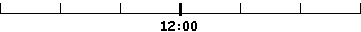For example, to calculate the elapsed time between 9:45 a.m. and 2:10 p.m., jumps might look like this: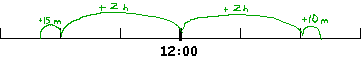With the timeline approach, it is easy to see that the elapsed time is 4 hours, 25 minutes.

## USING MENTAL TOOLS

There are many more tools we can place in a mental math tool bag. We want to give students a large tool bag, not just one tool. This article focuses on number line tools, because a mental number line is quite likely the most flexible, adaptable, and powerful tool. Sometimes, however, another mental strategy might prove easier. For example, when adding or subtracting two numbers with no regrouping involved, such as 345 + 612, its probably easier to add the hundreds (300 + 600), then the tens (40 + 10), and then the ones (5 + 2), than it is to jump along a huge mental number line. But the mental number line works well too: Start at 345, jump 600 to 945, jump 10 to 955, jump 2 to 957. Some strategies can be applied with or without visualization. To subtract 98 from 400, we can subtract 100 then add 2; on a mental number line, we can jump back 100 then jump forward 2. We also could add 2, and then subtract 100: 402 - 100. Either way, visualizing helps us understand how to adjust.

For younger students, perhaps the greatest challenge when doing mental math is holding several numbers in the mind at once. Breaking a number into chunks means understanding which chunks will be meaningful, mentally jumping with those chunks, and keeping track of the jumps to arrive at the correct answer. That ability comes with practice. In the meantime, doing mental math with visual training wheels helps build numerical flexibility and agility.

As students become more agile, they can take on larger mental computations, adding and subtracting numbers in the hundreds and even thousands, or skip-counting by any two-digit multiple. It's stimulating and empowering!

Janette Bobis, "The Empty Number Line: A Useful Tool or Just Another Procedure?" Teaching Children Mathematics, NCTM, April 2007, pp. 410-413